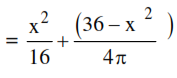# A wire of length 36 m is cut into two pieces,

Question:

A wire of length $36 \mathrm{~m}$ is cut into two pieces, one of the pieces is bent to form a square and the other is bent to form a circle. If the sum of the areas of the two figures is minimum, and the circumference of the circle is $\mathrm{k}$ (meter), then $\left(\frac{4}{\pi}+1\right) \mathrm{k}$ is equal to _______.

Solution:

Let $x+y=36$

$x$ is perimeter of square and $y$ is perimeter of circle side of square $=\mathrm{x} / 4$

radius of circle $=\frac{\mathrm{y}}{2 \pi}$

Sum Areas $=\left(\frac{\mathrm{x}}{4}\right)^{2}+\pi\left(\frac{\mathrm{y}}{2 \pi}\right)^{2}$For min Area :

$x=\frac{144}{\pi+4}$

$\Rightarrow$ Radius $=\mathrm{y}=36-\frac{144}{\pi+4}$

$\Rightarrow \mathrm{k}=\frac{36 \pi}{\pi+4}$

$\left(\frac{4}{\pi}+1\right) \mathrm{k}=36$